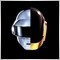• Overview
• Reviews (1)
• What's new

Stochastic Global

Description

A universal colored indicator Stochastic. The rising and falling lines and the levels of the indicator can be colored.

The indicator can be calculated as the standard one on the basis of a price or as a derivative on the basis of the following nineteen other indicators included in the standard package of the MetaTrader 4 terminal:

1. AD - an indicator of accumulation and distribution of volume.
2. OBV - On Balance Volume.
3. Volume - tick volume indicator.
4. AC - indicator of acceleration.
5. AO - Williams' oscillator.
6. BullsPower.
7. BearsPower.
8. BullsPower-BearsPower - the difference of the bulls power and bears power.
9. Force - Force Index indicator.
10. MFI - Money Flow Index.
11. ATR - Average True Range.
12. Momentum.
13. RSI - Relative Strength Index.
14. Moving Average.
15. CCI - Commodity Channel Index.
16. ADX - Average Directional Movement indicator.
17. DeMarker.
18. Standard Deviation.
19. OsMA - moving average of oscillator.
20. MACD - moving average convergence/divergence.

Price is used by default.

Purpose

The indicator can be used for manual or automated trading in an Expert Advisor. Values of indicator buffers of the double type can be used for automated trading:

1. The main line of the indicator - buffer 5.
2. The rising line of the indicator - buffer 0.
3. The falling the line indicator - buffer 1.
4. The signal line of the indicator - buffer 2.

Inputs

1. Calc_Method - the index of the indicator, based on which the Stochastic will be calculated (from 0 to 19). By default, i.e. more than 19 - the standard one based on Low/High prices, with Simple averaging.
2. KPeriod - external parameters of Stochastic.
3. DPeriod - external parameters of Stochastic.
4. Slowing - external parameters of Stochastic.
5. Periods - the period for calculating the basic indicator, based on which Stochastic will be calculated.
6. Fast_Period - the fast MA period of the basic indicator (for example MACD), based on which Stochastic will be calculated.
7. Slow_Period - the slow MA period of the basic indicator.
8. Signal_Period - the signal line period of the basic indicator.
9. MA_Method - averaging method for the MA of the basic indicator:
1. MODE_SMA - 0.
2. MODE_EMA - 1.
3. MODE_SMMA - 2.
4. MODE_LWMA - 3.
10. Signal_Method - The averaging method of the signal line of the Stochastic:
1. MODE_SMA - 0.
2. MODE_EMA - 1.
3. MODE_SMMA - 2.
4. MODE_LWMA - 3.
11. Applied_Price - the used price of the basic indicator:
1. PRICE_CLOSE - 0.
2. PRICE_OPEN - 1.
3. PRICE_HIGH - 2.
4. PRICE_LOW - 3.
5. PRICE_MEDIAN - 4.
6. PRICE_TYPICAL - 5.
7. PRICE_WEIGHTED - 6.
12. Mode - the index of the line (MAIN - 0 or SIGNAL - 1) of the basic indicator, based on which the Stochastic will be calculated.
13. Shift - the sift o the basic indicator relative to the price chart.4954
2014.06.20 07:20

A great oscillator. Very responsive s and seems to have practically no lag.

Version 1.22 2014.10.23
User's interface has been improved.